Home | | Electronic Circuits I | Midband analysis of BJT Single Stage Amplifiers

# Midband analysis of BJT Single Stage Amplifiers

Consider the basic amplifier circuit. To form a transistor amplifier only is is necessary to connect an external load and signal source along with proper biasing.

Midband analysis of BJT Single Stage Amplifiers:

Consider the basic amplifier circuit. To form a transistor amplifier only is is necessary to connect an external load and signal source along with proper biasing.We can replace the transistor circuit as shown in the following figure.Let us analyze the hybrid model to find current gain, input resistance, voltage gain and output resistance.

Current gain (Ai):

It is defined as the ratio of output to input current. It is given by,Here IL and I2 are equal in magnitude but opposite in sign. IL = -I2

From above circuitCurrent gain (AIS):

It is given by,From above figure, using current divider rule,Input Impedance (Zi):

Ri is the input resistance looking into the amplifier input terminals ( 1, 1’). It is given by,From the input circuit,Substituting V2 = -I2RL = Ai I1 RL in the above equation,Dividing numerator and denominator by RL we get,From this equation, note that the input impedance is a function offload impedance.

Voltage gain (Av):

It is the ratio of output voltage to input voltage. It is given by,By substituting V2 = -I2RL = Ai I1 RLVoltage gain (Avs):

It is voltage gain including the source. It is given by,From above figure, applying potential divider rule, then we get,Substituting the value of V1/Vs in the equation of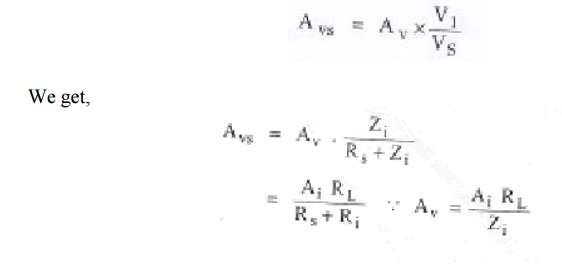It is the ratio of output current to output voltage. It is given by,Dividing above equation by V2, We get,From transistor amplifier in h-parameter model circuit, with Vs = 0,

RsI1 + hiI1 +hrV2 = 0

(Rs + hi) I 1 = -hr V2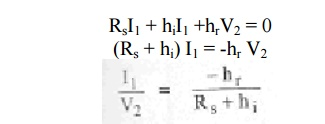Substituting the value of I1/V2 from above equation in the equation of Yo. We obtain,From this equation, note that the output admittance is a function of source resistance.

Power gain (Ap):

It is the ratio of average power delivered to the load to the input power. Output power is given as,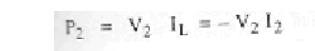Since the input power is P1 = V1I1

The operating power gain Ap of the transistor is given as,Relation between Avs and AIS

From equation,Taking ratio of above two equations we get,Method for analysis of a transistor circuit:

The analysis of transistor circuits for small signal behaviour can be made by following simple guidelines. These guidelines are,

1.     Draw the actual circuit diagram

2.     Replace coupling capacitors and emitter bypass capacitor by short circuit

3.     Replace D.C. source by a short circuit

4.     Mark the points B, E, C on the circuit diagram and locate these points as the start of the equivalent circuit

5.     Replace the transistor by its h-parameter model

Problem 1:

For the common base circuit shown in figure, transistor parameters are hib = 22Ω,

hfb = -0.98, hob = 0.49µA/V, hrb = 2.9*10 -4. Calculate the values of input resistance, output resistance, current gain and voltage gain for the given circuit.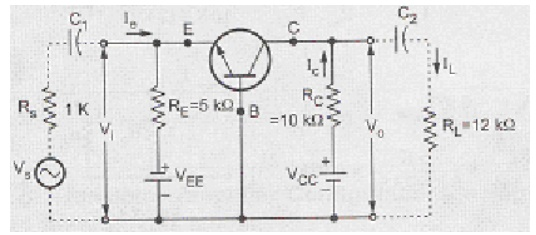Solution:

Change the given figure into h-parameter equivalent model.Ro' = Ro || RL’ = 1.21M || 5.45K = 5.425KΩ

Problem 2:

Consider a single stage CE amplifier with Rs = 1KΩ, RL = 1.2KΩ. Calculate Ai, Ri,  Av, Ais, power gain and Ro if hie = 1.1k, hre = 2.5*10-4, hfe = 50 and hoe = 25µA/V.

Solution:Problem 3:

Consider a single stage CE amplifier with Rs = 1k, R1 = 50k, R2 = 2k, Rc = 2k, RL =  2K, hie = 1.1k, hoe = 25µA/V, hfe = 50 and hre = 2.5*10-4 as shown in the figure.

Find Ai, Ri, Av, Ai, Avs and Ro.Solution:

Since hoe RL' = 25*10-6*(2K || 2K) = 0.25, which is less than 0.1, so use approximate analysis.

Consider the simplified hybrid model for the given circuit.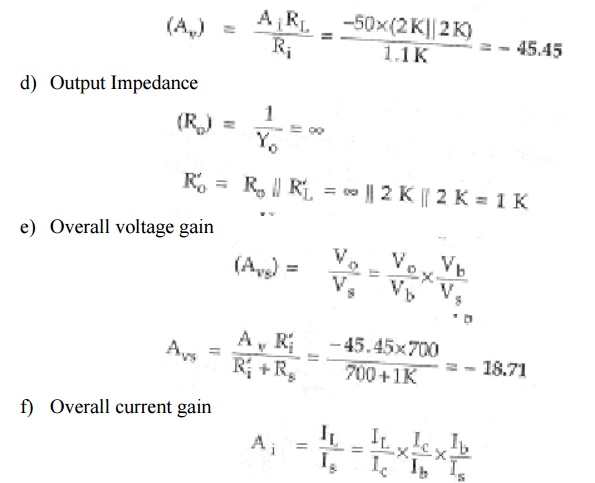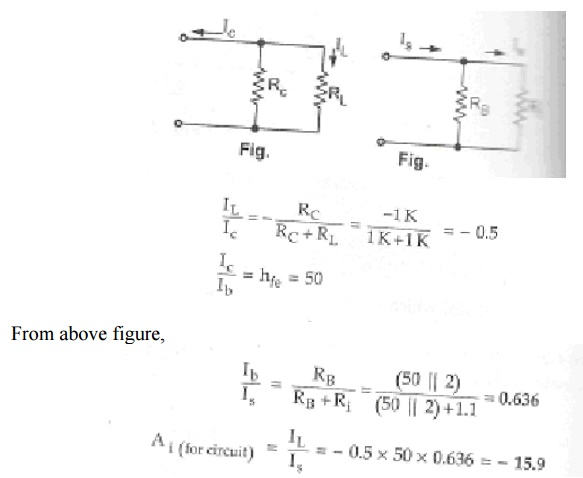Comparison of Transistor Configurations:Study Material, Lecturing Notes, Assignment, Reference, Wiki description explanation, brief detail
Electronic Circuits : BJT Amplifiers : Midband analysis of BJT Single Stage Amplifiers |